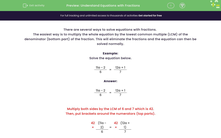# Solve Equations with Fractions

In this worksheet, students will learn how to solve equations with fractions.Key stage:  KS 3

Curriculum topic:   Algebra

Curriculum subtopic:   Solve Linear Equations (One Variable)

Popular topics:   Algebra worksheets

Difficulty level:#### Worksheet Overview

There are several ways to solve equations with fractions.

The easiest way is to multiply the whole equation by the lowest common multiple (LCM) of the denominator (bottom part) of the fraction. This will eliminate the fractions and the equation can then be solved normally.

Example:

Solve the equation below.

 11a - 2 = 12a + 1 6 7

 11a - 2 = 12a + 1 6 7

Multiply both sides by the LCM of 6 and 7 which is 42.

Then, put brackets around the numerators (top parts).

 42 × (11a - 2) = 42 × (12a + 1) 6 7

Reduce the fractions:

 7 42 × (11a - 2) = 6 42 × (12a + 1) 6 7

Simplify:

7 (11a - 2) = 6 (12a + 1)

Multiply out the brackets:

77a - 14 = 72a + 6

77a - 14 + 14 = 72a + 6 + 14

Simplify:

77a = 72a + 20

Subtract 72a from both sides:

77a -72a = 72a + 20 - 72a

Simplify:

5a = 20

Divide both sides by 5:

5a ÷ 5 = 20 ÷ 5

Simplify:

a = 4

Ensure you have a pen and paper so that you can write down your working out. This will enable you to spot any errors and, even if you get the final answer incorrect in your exams you may still gain valuable marks.

### What is EdPlace?

We're your National Curriculum aligned online education content provider helping each child succeed in English, maths and science from year 1 to GCSE. With an EdPlace account you’ll be able to track and measure progress, helping each child achieve their best. We build confidence and attainment by personalising each child’s learning at a level that suits them.

Get started